### Computational Resources

Let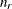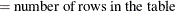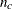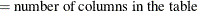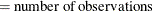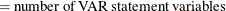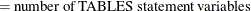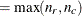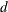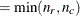For TABLES statement input, more than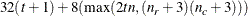bytes of array space are required.

For VAR statement input, more than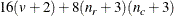bytes of array space are required.

#### Memory

The computational resources formulas are underestimates of the amounts of memory needed to handle most problems. If you use a utility data set, and if memory could be used with perfect efficiency, then roughly the stated amount of memory would be needed. In reality, most problems require at least two or three times the minimum.

PROC CORRESP tries to store the raw data (TABLES input) and the contingency table in memory. If there is not enough memory, a utility data set is used, potentially resulting in a large increase in execution time.

#### Time

The time required to perform the generalized singular value decomposition is roughly proportional to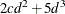. Overall computation time increases with table size at a rate roughly proportional to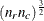.